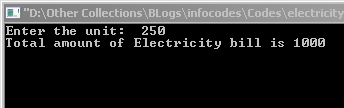# Electricity Bill Calculation using C a Program

Program Abstraction:      Here you can calculate your electricity bill using a C program with bill constraints.

Calculating electricity bill using C program is a common question in C. We have already learnt the working of If-else in a previous lesson. So this program use the IF-Else Ladder condition.

Here there are two types of program to find the amount of electricity bill.

## Type 1: Calculate Electricity bill using given units used.

Here the bill amount is calculated according to this condition:

If unit used is more than 500, then amount will be calculated by 5 rupees for each unit. Like this:
If Unit >350 and <500, then rupees 4.5 per units
If Unit >200 and <350, then rupees 4 per units
If Unit >100 and <200, then rupees 3.5 per units
If Unit <100, then rupees 3 per units

`#include <stdio.h>`

#include <conio.h>
void main()

{

int unit;

int amount;

printf(“Enter the unit: “);

scanf(“%d”,&unit);

if(unit>500)

{

amount = unit * 5;

}

else if(unit>350)

{

amount = unit * 4.5;

}

else if(unit>200)

{

amount = unit * 4;

}

else if(unit>100)

{

amount = unit * 3.5;

}

else if(unit<=100)

{

amount = unit * 3;

}

printf(“Total amount of Electricity bill is %d “, amount);

getch();

}

In above code, we calculate amount from units upper top to bottom order(500 to 100). So IF contains only one condition, for example: instead of (unit>350 && unit<500) we use only (unit>350), because, unit >500 is already checked.

Sample Output:## Type 2: Calculate Electricity bill using given old meter and new meter reading.

`#include <stdio.h>`

#include <conio.h>

void main()
{
int unit;
int amount;

printf(“Enter the old meter reading : “);
printf(“Enter the new meter reading : “);

if(unit<=100)
{
amount = unit * 3;
}
else if(unit>100 && unit <=200)
{
amount = unit * 3.5;
}
else if(unit>200 && unit<=350)
{
amount = unit * 4;
}
else if(unit>350 && unit <=500)
{
amount = unit * 4.5;
}
else if(unit>500)
{
amount = unit * 5;
}
printf(“Total amount of Electricity bill is %d”,amount);
getch();
}

Here we find the units used from new meter reading and old meter reading.  And we start to check the condition from least number (100), so use both conditions in a single if condition. e.g.: (unit>200 && unit<=350))

Sample Output: